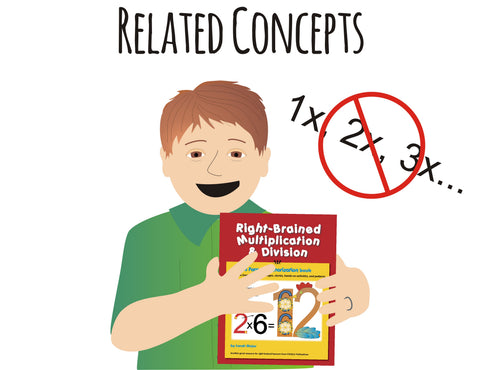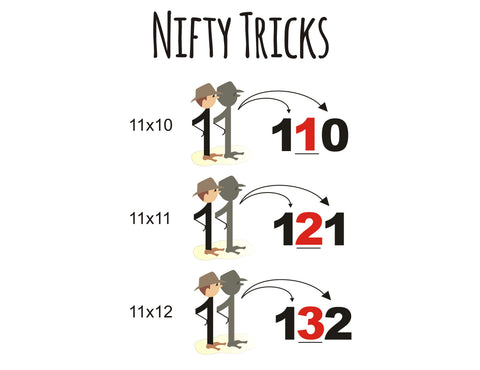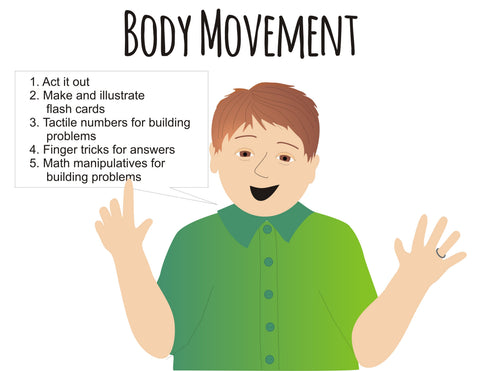Five Right-Brained Tools For Teaching Multiplication & Division

# Five Right-Brained Tools For Teaching Multiplication & Division

Thank you for your interest in Right-Brained Multiplication & Division! This book is exciting because it teaches using 5 powerful tools.

## The 5 Tools

1. Visuals
2. Personification
3. Pattern Discovery
4. Story
5. Body MovementVISUALS are captured instantly. They are used everywhere to show what we are explaining. In the visuals of math facts, the numbers are included in the visuals. Visuals show students what the problem means and give them a mental image of the problem.We use PERSONIFICATION & STORIES to teach rules. For example, the book begins with Zeroman. With a POOSH of his wand, everything he multiplies or divides turns to a zero. It is a simple as that.Most children love finding PATTERNS in math – and discovering patterns makes it easy to understand how to solve problems and to remember math procedures.

Mr. 10 always tosses a ring to any number that comes through his door. So 10 times any number will be that number with a “ring” after it.Many math concepts are related. With this idea in mind, Sarah changed the order of learning from 1’s then 2’s then 3’s to an order that allows for related concepts to be learned together.

For example, after students learn the 1’s table in which Agent 1 says, “Just be yourself, Dahling!” we move to the 11’s table because 11’s follow many of the same patterns as the 1’s table does.

1 times 5 is that same number.

11 times 5 is two of that same number.This book also contains many nifty tricks to help students solve difficult math problems without memorizing facts. For example 11 times a double digit number is easily solved if you add the two digits of the number and put their sum between them.

Right-Brained Multiplication & Division teaches both processes at one time so children can see the relationship that exists between Multiplication and Division.There are also hands-on activities in each chapter that add to that all important element of BODY MOVEMENT and provide a tangible way to practice the concepts students are learning.

Let’s take the stress out of math! Pick up your copy of Right-Brained Multiplication & Division today!